# Weierstrass theorem

There are a number of theorems named after Karl Theodor Wilhelm Weierstrass (1815-1897).

### Infinite product theorem

Weierstrass' infinite product theorem : For any given sequence of points in the complex plane,(1)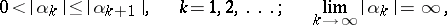there exists an entire function with zeros at the points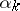of this sequence and only at these points. This function may be constructed as a canonical product:(2)

whereis the multiplicity of zero in the sequence (1), and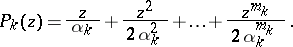The multipliersare called Weierstrass prime multipliers or elementary factors. The exponents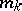are chosen so as to ensure the convergence of the product (2); for instance, the choice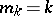ensures the convergence of (2) for any sequence of the form (1).

It also follows from this theorem that any entire functionwith zeros (1) has the form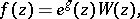whereis the canonical product (2) and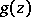is an entire function (see also Hadamard theorem on entire functions).

Weierstrass' infinite product theorem can be generalized to the case of an arbitrary domain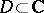: Whatever a sequence of points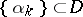without limit points in, there exists a holomorphic functioninwith zeros at the pointsand only at these points.

The part of the theorem concerning the existence of an entire function with arbitrarily specified zeros may be generalized to functions of several complex variables as follows: Let each pointof the complex space,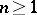, be brought into correspondence with one of its neighbourhoods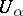and with a function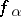which is holomorphic in. Moreover, suppose this is done in such a way that if the intersectionof the neighbourhoods of the pointsis non-empty, then the fraction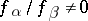is a holomorphic function in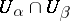. Under these conditions there exists an entire functioninsuch that the fraction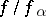is a holomorphic function at every point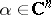. This theorem is known as Cousin's second theorem (see also Cousin problems).